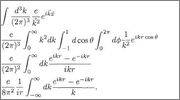# Imaginary sinusoidal exponential

• I
• spaghetti3451

#### spaghetti3451

I need to convince myself that

##e^{ikr\text{cos}\ \theta}=\frac{\text{sin}\ \theta}{kr}##.

Cannot be true, the left side is complex whereas the right side is real.

Sorry, there was a typo.

##\text{exp}({ikr\text{cos}\ \theta}) = \frac{\text{exp}(ikr)-\text{exp}(-ikr)}{2ikr}##?

What do you know about the Euler formula for a complex exponential ##e^{i\phi}##?

The Euler formula is ##e^{i\phi}= \text{cos}\ \phi + \text{sin}\ \phi##.

I don't see how ##\text{exp}(ikr\text{cos}\ \theta) = \frac{\text{sin}(kr)}{kr}##.

The Euler formula is ##e^{i\phi}= \text{cos}\ \phi + \text{sin}\ \phi##.
It's ##e^{i\phi}= \text{cos}\ \phi + i\text{sin}\ \phi##.
I don't see how ##\text{exp}(ikr\text{cos}\ \theta) = \frac{\text{sin}(kr)}{kr}##.
Indeed, I too, do not see how that can be true.

The following is taken from page 39 of Matthew Schwartz's 'Introduction to Quantum Field Theory.' My above posts refer to the third and fourth lines of the following calculation:a

Would you please have a look and let me know what I'm missing in the previous posts?

$$\int_{-1}^1 d(\cos \theta) e^{ikr\cos \theta} = \frac{1}{ikr}e^{ikr\cos \theta}\Big|_{-1}^1 = \frac{e^{ikr}-e^{-ikr}}{ikr}$$
Then in the third line you have ##\frac{\sin kr}{k}## as the integrand to be integrated from 0 to infiinity. The function ##\frac{\sin kr}{k}## is an even function, thus
$$\int_0^\infty \frac{\sin kr}{k} \, dk = \frac{1}{2} \int_{-\infty}^\infty \frac{\sin kr}{k} \, dk$$

•spaghetti3451
Thanks! I feel like a total idiot now!